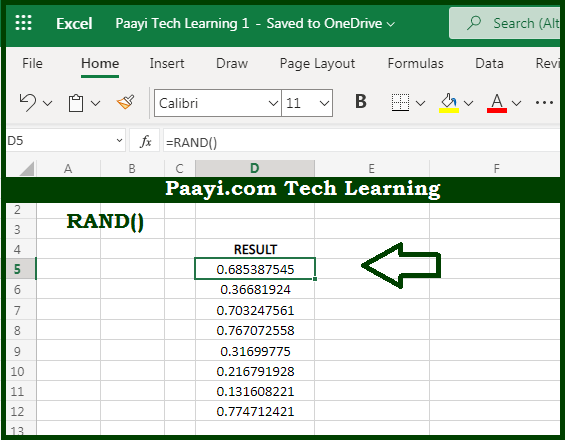# Learn How to Use Microsoft Excel RAND Function

Written by | 0 Comments | 623 Views

In this article, you will learn how to use the Microsoft Excel RAND function and its prime function in Microsoft Excel. You will also get to know the Microsoft Excel RAND function return value and syntax with the help of some examples.

Microsoft Excel RAND Function

The main function of the Microsoft Excel RAND function is to get the random number between 0 and 1. That implies, with the help of the RAND function you can able to return the random numbers between 0 and 1 as a result. Let's say for example, =RAND() will return a result in the form of a number like 0.422245717. The RAND function recalculates when a worksheet is opened or changed. So, with the help of the RAND function, you can able to get the random number between 0 and 1.

Return Value of RAND Function

The return value will be the number between 0 and 1.

Syntax of RAND Function

=RAND( )

## How to Use Microsoft Excel RAND Function?So we know that Microsoft Excel RAND function you can able to get the random number between 0 and 1. That implies, with the help of the RAND function you can able to return the random numbers between 0 and 1 as a result. Let's say for example, =RAND() will return a result in the form of a number like 0.422245717. The RAND function recalculates when a worksheet is opened or changed. So, with the help of the RAND function, you can able to get the random number between 0 and 1.